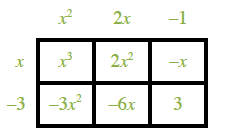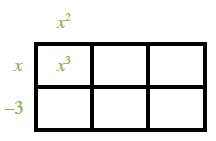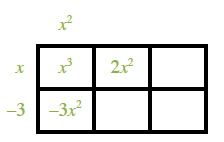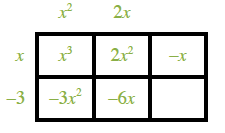8-138.

Missing problem for Book CCA2 => Chapter Ch8 => Lesson 8.3.2 => Problem 8-138

Created from orphaned homework help problem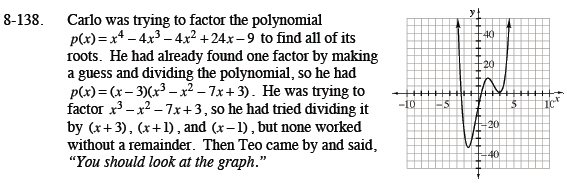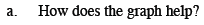It shows that x = 3 is a double root, so (x − 3) is a repeated factor.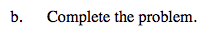Divide x3x2 − 7x + 3 by (x − 3).

Now use the Quadratic Formula to solve for x.

$x = 3, -1 \pm \sqrt{2}$